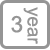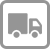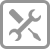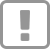• 产品详情

20m³大吸力，给您“净”致厨房

--捕风猎手，智享轻奢，开启侧吸新体验

ED805 侧吸式油烟机

^^^^^^^^^^^^^^^^^^^^^^^^^^^^^^^^^^^^^^^^^^^^^^^^^^^^^^^^^^^^^^^^^^^^^^^^^^^^^^^^^^^

3200cm的“巨幕拢烟屏”，能带来深达16000cm³的有效拢烟区，形成一个超大倒锥漏斗负压区，“近距劲吸”、“深腔拢烟”与“屏随烟动”三大核心技术智能配合，对油烟进行智能操控，实现油烟全吸走。

^^^^^^^^^^^^^^^^^^^^^^^^^^^^^^^^^^^^^^^^^^^^^^^^^^^^^^^^^^^^^^^^^^^^^^^^^^^^^^^^^^^

>

Professional security care for a better life

【享受服务】